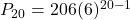## Find P 20 of the following arithmetic sequence: 206, 212, 218, 224- State: Po = d = P20 =

Question

Find P 20 of the following arithmetic sequence: 206, 212, 218, 224….
State:
Po =
d =
P20 =

in progress 0
2 weeks 2021-08-29T00:16:20+00:00 1 Answers 0 views 0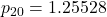P₀ = 206 and difference d = 6

Step-by-step explanation:

Step(i):-

Given that the arithmetic sequence

206,212,218,224,…..

P₀ = 206 and difference d = 212-206 = 6

The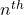term of the sequence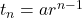Step(ii):-

Theterm of the sequence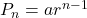Put n=20